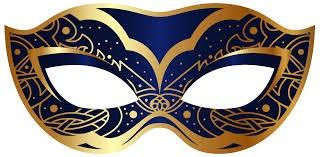Howdy, Stranger!

We are about to switch to a new forum software. Until then we have removed the registration on this forum.

Create an Image Mask On Top of a Live Feed

edited March 2017 in Kinect

Hey all!

So for this code I'm trying to create a code that will use a live webcam feed and face tracking to put an image on top of the tracked face. After this point, I want to press a key ('n' in the code I posted below) and have it switch to a different picture. Right now as a base code I have processing's "LiveFaceTracking" example in my code. Any help you guys could give me would be greatly appreciated!

import gab.opencv.*;
import processing.video.*;
import java.awt.*;
PImage WD;
PImage GJ;

Capture video;
OpenCV opencv;

void setup() {
size(640, 480);
video = new Capture(this, 640/2, 480/2);
opencv = new OpenCV(this, 640/2, 480/2);
video.start();

}

void draw() {
scale(2);

image(video, 0, 0 );

noFill();
stroke(0, 255, 0);
strokeWeight(3);
Rectangle[] faces = opencv.detect();
println(faces.length);

for (int i = 0; i < faces.length; i++) {
println(faces[i].x + "," + faces[i].y);
rect(faces[i].x, faces[i].y, faces[i].width, faces[i].height);
}
}

void captureEvent(Capture c) {
}

void keyPressed(){
if(key = 'n'){
Tagged:

• When you say "image mask" do you mean a picture of a mask, with transparency:Or do you mean an "image mask":

Examples of applying a mask() to an image: https://forum.processing.org/two/discussion/comment/78559/#Comment_78559

• I mean an image of a mask with transparency! Sorry about that I should've been extra clear.

2. Instead of drawing a rectangle with rect(), draw an image with image()
3. image() takes location arguments -- try starting with the ones you are using for your rects image(maskimg, faces[i].x, faces[i].y).

Now you need to think about alignment, centering, tilting, etc. -- faces.SOMETHING might give you more information for positioning.

faces is what you got from opencv.detect() -- look up opencv.detect to see what it creates, and what you can do with that information!

• If I could, I have one more question. Here is what I have for my code now. The biggest issue that I have now is that, in theory, it should be loading the second image when "image1" is false but it isn't. If you can see something that I'm missing, I would really appreciate the help.

import gab.opencv.*;
import processing.video.*;
import java.awt.*;
PImage WD;
PImage GJ;
boolean image1;

Capture video;
OpenCV opencv;

void setup() {
size(640, 480);
video = new Capture(this, 640/2, 480/2);
opencv = new OpenCV(this, 640/2, 480/2);
video.start();

image1 = true;
}

void draw() {
scale(2);
image(video, 0, 0 );
Rectangle[] faces = opencv.detect();
println("image1" + image1);

if (image1 == true){
for (int i = 0; i < faces.length; i++) {
image(GJ,faces[i].x, faces[i].y, faces[i].width, faces[i].height);}

if(image1 == false){
for (int i = 0; i < faces.length; i++) {
image(WD,faces[i].x, faces[i].y, faces[i].width, faces[i].height);}

}}}

void captureEvent(Capture c) {
}

void keyPressed(){
if(key == 'o'){
image1 = true;
}
if(key == 'p'){
image1 = false;
}}

• @2lbrez16 -- You have messed up your if statements -- they don't do what you think they do. Highlight your code in Processing and press Command-T to fix the spacing and make it easier to read.

You will now see that you are only checking if it is "false" after you have already found it is "true" -- which is impossible.

So fix that.

• I'm not sure how I would fix that. But I would need to fix it even if I'm trying to load a second mask over the face when it is false?

• Did you actually follow my instructions and look at the code?

• You think you wrote this:

• if (image1 == true)
• if (image1 == false)

...but what you wrote is this:

• if (image1 == true)
• now, if (image1 as also false)
• WD mask (this will never happen, because a true thing is never also false)

So, fix the { } to make it like the first one.

• I'm sorry, I did but I'm very new to this so I'm not exactly sure what I'm supposed to be looking for.

• I feel bad for asking so many questions. I've been staring at the code and trying to look it up and see if I can figure it out but I can't seem to find anything that makes sense. I'm just not sure exactly what to change about the if statements.

• You need to understand how if statements work.

Here is an example you need to be looking for:

This (correct) -- two checks, next to each other:

if (keyPressed == true){
print("true");
}
if(keyPressed == false){
print("false")
}

...and not your problem, which is like this (wrong) -- one check inside the other one:

if (keyPressed == true){
print("true");
if(keyPressed == false){
print("false")
}
}

If you don't understand the difference, try them out in a sketch. Once you figure it out, you will be able to fix your sketch. You are checking the wrong way. Do it the correct way. You can also simply use if { } else { }.

• Did you find a solution to this? Would you be able to post your code? Thanks

• @lcjmiller -- a solution to which part of the the thread?